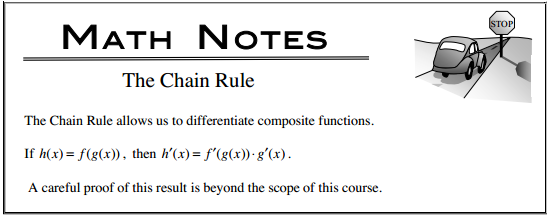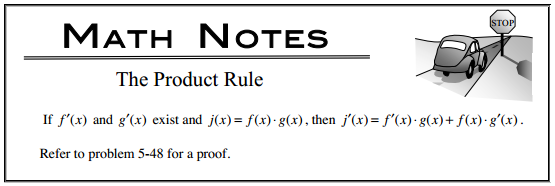### Home > CALC > Chapter 6 > Lesson 6.1.2 > Problem6-24

6-24.

First, decide if differentiating the following expressions requires the Product Rule, the Quotient Rule, the Chain Rule, or a combination of these rules. Then, find the following derivatives.

1. $\frac { d } { d t } [ f ( t ^ { 2 } ) ]$1. $\frac { d } { d x } [ x \cdot h ( x ) ]$1. $\frac { d } { d t } [ t \cdot h ( t ^ { 2 } ) ]$